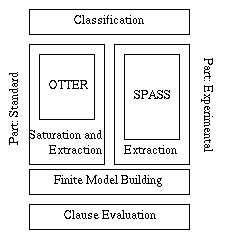# The Incredible Logic Tool

## Abstract

Well established fields in Automated Deduction focus on algorithms for the detection of unsatisfiability (or, equivalently, validity) of sets of clauses (first-order formulas). However, these algorithms are rather useless if the input clause set turns out to be satisfiable: Only if the system terminates, the user can recognize the existence of a counter-example to the tested specification. Which one, he/she can only guess. In this paper we present TILT an automated deduction system designed to overcome this lack of expressiveness.
Assume a statement or specification expressed as a first-order formula F Well established fields in Automated Deduction focus on algorithms for the detection of unsatisfiability of clause sets C, sat-equivalent to not F. However, it is at least thinkable that F does not hold in all possible worlds. Then, usually, the only information one can extract from the output---if the system terminates at all---is: There exists a counter-example to statement F. Which one, the user can only guess.

We express the idea more formally:
Assume a deduction system I based on resolution. To prove the validity of F, not F is transformed into a sat-equivalent clause set C, To keep our argumentation simple, we assume C to be decidable by I If I is applied on C, then it terminates with a saturated set of clauses C' not containing the empty clause. Provided I is refutationally complete, C' is consistent. Hence, the set C represents a (possibly infinite) class of counter-examples, but it is usually not possible to extract one of these models of C' directly. In most cases it is not even possible to extract an `understandable' model representation of one of these models.
The goal of our approach to Automated Model Building is to transform C' into a representation of an (infinite) Herbrand model of \C. We call this representation model representation.

We motivate this by giving an example:
No hungry cat gazes at the moon. Some cats gazing at the moon are in love. Conclude that some cats which are in love are hungry.
We want to know whether the conclusion is logically justified or not.

Let "H(x)" stand for "x is a hungry cat", "G(x)" for "cat x gazes at the moon", and "L(x)" for "cat x is in love". Now, we encode our problem in first-order logic:

F <-> [(forall x)( H(x) -> not G(x)) and (exists x)( G(x) and L(x))] -> (exists x)( L(x) and H(x)).

To apply resolution, we have to transform not F into a clause set

C= {not H(x) or not G(x), G(a), L(a), not L(x) or not H(x) }

It is easy to see that any reasonable resolution refinement terminates on C, without inferring the empty clause. If we want to correct F such that it becomes valid, the counter-example (assuming, we can actually construct one), can serve as guiding data. With the methods employed in TILT the following Herbrand model I = { not H(a), G(a), L(a) } is derivable. This models describes a word in which exists exactly one cat a, this cat is in love, free of hunger and gazing at the moon.

There are arbitrarily many possibilities to modify a statement so that it becomes valid. If we decide that our premises are correct, we have to reconsider the conclusion:
No hungry cat gazes at the moon. Some cats gazing at the moon are in love. Conclude that some cats which are in love are {\em not} hungry.

We denote the corresponding formula as F'. Applying resolution to the new clause set C', sat-equivalent to not F', we derive the empty clause. Hence, the new conclusion is justified. We call this approach to Automated Model building resolution-based.
The deduction system TILT implements the recent results of the Vienna Group. TILT embodies three components
• Classification
The Classification component tests the input clause set C for membership in safe classes.
• Model Building
If C turns out to be satisfiable, the information achieved in the Classification component is used to generate a model representation for C. This component splits into two independent parts: a standard and an experimental part.
• Clause Evaluation
The obtained model representation is applied to evaluate general clauses based on the same signature as C.
We aim at an integrated deduction system employing several theorem provers such that the user is provided with full control over the strategies of each prover, but on the other hand obtains as much information in the case the prover fails on the input clause. The information has to be provided in a form easily to grasp. TILT is our first step in this direction.

The overall structure of TILT is best presented by a picture